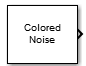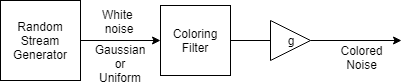Colored Noise

Generate colored noise signal

• Library:
• DSP System Toolbox / Sources

•Description

The Colored Noise block generates a colored noise signal with a power spectral density of 1/|f|α over its entire frequency range. The inverse power spectral density component, α, can be any value in the interval [-2 2]. The type of colored noise the block generates depends on the Noise color option you choose in the block dialog box. When you set Noise color to custom, you can specify the power density of the noise through the Power of inverse frequency parameter.

Ports

Output

expand all

Colored noise output signal. The size and data type of the signal depend on the values of the Number of output channels, Number of samples per output channel, and Output data type parameters.

Data Types: single | double

Parameters

expand all

Color of the noise the block generates. You can set this parameter to:

• pink — Generates pink noise. This option is equivalent to setting Power of inverse frequency to 1.

• white — Generates white noise (flat power spectral density). This option is equivalent to setting Power of inverse frequency to 0.

• brown — Generates brown noise. Also known as red or Brownian noise. This option is equivalent to setting Power of inverse frequency to 2.

• blue — Generates blue noise. Also known as azure noise. This option is equivalent to setting Power of inverse frequency to -1.

• purple — Generates violet (purple) noise. This option is equivalent to setting Power of inverse frequency to -2.

• custom — Specify the power density of the noise using the Power of inverse frequency parameter.

Inverse power spectral density component, α, specified as a real-valued scalar in the interval [-2 2]. The inverse exponent defines the power spectral density of the random process by 1/|f|α. The default value of this property is 1. When Power of inverse frequency is greater than 0, the block generates lowpass noise, with a singularity (pole) at f= 0. These processes exhibit long memory. When Power of inverse frequency is less than 0, the block generates highpass noise with negatively correlated increments. These processes are referred to as antipersistent. In a log-log plot of power as a function of frequency, processes generated by the Colored Noise block exhibit an approximate linear relationship, with the slope equal to –α.

Dependencies

To enable this parameter, set Noise color to custom.

Select the parameter to make the output bounded between +1 and −1.

When you select the parameter, the internal random source that generates the noise is uniform. If Noise color is set to white, there is no color filter applied to the output of the random source. The output is uniform noise of amplitude between +1 and −1. If Noise color is set to any other option, then a coloring filter is applied to the output of the random source, followed by a gain that ensures the absolute maximum output never exceeds 1.

When you do not select the parameter, the internal random source is Gaussian. The output is not bounded.

Number of output channels, specified as a positive integer scalar. This parameter defines the number of columns in the generated signal.

Data type of the output specified as double or single.

Number of samples in each frame of the output signal, specified as a positive integer scalar. This parameter defines the number of rows in the generated signal.

Sample time of the output signal, specified as a positive scalar in seconds.

Initial seed of the random number generator algorithm, specified as a real-valued positive integer scalar.

Type of simulation to run. You can set this parameter to:

• Interpreted execution

Simulate model using the MATLAB® interpreter. This option shortens startup time.

• Code generation

Simulate model using generated C code. The first time you run a simulation, Simulink® generates C code for the block. The C code is reused for subsequent simulations, as long as the model does not change. This option requires additional startup time.

Block Characteristics

 Data Types double | single Multidimensional Signals No Variable-Size Signals No

expand all

Algorithms

The figure shows the overall process of generating the colored noise.The random stream generator produces a stream of white noise that is either Gaussian or uniform in distribution. A coloring filter applied to the white noise generates colored noise with a power spectral density (PSD) function given by:

$S\left(f\right)=\frac{L\left(f\right)}{|f{|}^{\alpha }}$

When α, the inverse frequency power, equals 0, no coloring filter is applied to the output of the random stream generator. If the bounded option is enabled, the output is uniform white noise with amplitude between +1 and −1. If the bounded output is not enabled, the output is a Gaussian white noise and the values are not bounded between +1 and −1. If α is set to any other value, then a coloring filter is applied to the output of the random stream generator. If the bounded output option is enabled, a gain g is applied to the output of the coloring filter to ensure that the absolute maximum output never exceeds 1.

For details on colored noise processes and how the value of α affects the PSD of the colored noise, see Colored Noise Processes.

When the inverse frequency power α is positive, the colored noise is generated using an auto regressive (AR) model of order 63. The AR coefficients are:

$\begin{array}{l}{a}_{0}=1,\\ {a}_{k}=\left(k-1-\frac{\alpha }{2}\right)\frac{{a}_{k-1}}{k},\text{\hspace{0.17em}}\text{\hspace{0.17em}}\text{\hspace{0.17em}}k=1,2,\dots ,63\end{array}$

Pink and brown noises are special cases, which are generated from specially tuned SOS filters of orders 12 and 10, respectively. These filters are optimized for better performance.

When the inverse frequency power α is negative, the colored noise is generated using a moving average (MA) model of order 255. The MA coefficients are:

$\begin{array}{l}{b}_{0}=1,\\ {b}_{k}=\left(k-1+\frac{\alpha }{2}\right)\frac{{b}_{k-1}}{k},\text{\hspace{0.17em}}\text{\hspace{0.17em}}k=1,2,\cdots ,255\end{array}$

Purple noise is generated from a first order filter, B = [1 −1].

The coloring filters applied (except pink, brown, and purple) are detailed on pp. 820–822 in .

 Beran, J., Feng, Y., Ghosh, S., and Kulik, R. Long-Memory Processes: Probabilistic Properties and Statistical Methods. Springer, 2013.

 Kasdin, N.J. "Discrete Simulation of Colored Noise and Stochastic Processes and 1/fα Power Law Noise Generation". Proceedings of the IEEE®. Vol. 83, No. 5, 1995, pp. 802–827.

DSP System Toolbox Documentation